# 背景

• 搜索场景中，只有被当前投放策略排到前面的商品，才会获得曝光机会，从而形成监督学习的正负样本，而曝光出来的商品，只占总的召回商品中的很小一部分，训练样本是高度受当前模型的bias影响的。
• 监督学习的损失函数，和业务关注的指标之间，存在着不一致性
• 用户的搜索、点击、购买行为，是一个连续的序列决策过程，监督模型无法对这个过程进行建模，无法优化长期累积奖赏。

# 基于强化学习的实时搜索排序调控

## 背景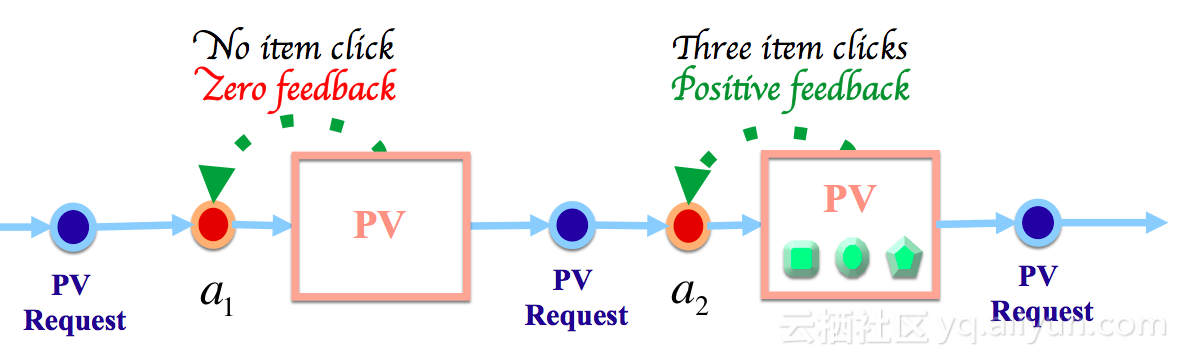## 问题建模

### 强化学习简介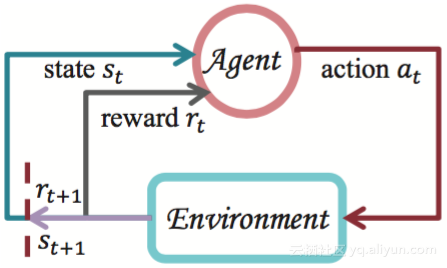$${\pi}^* = argmax_{\pi} \mathbb{E}_{\pi} \{ \sum_{k=0}^{\infty} \gamma^k r_{t+k} | s_t = s \}, \forall s \in S, \forall t \geq 0.$$

$$V^*(s) = \max_{\pi} \mathbb{E}_{\pi} \{ \sum_{k=0}^{\infty} \gamma^k r_{t+k} | s_t = s \}, \forall s \in S, \forall t \geq 0$$

$$Q^*(s,a) = \max_{\pi} \mathbb{E}_{\pi} \{ \sum_{k=0}^{\infty} \gamma^k r_{t+k} | s_t = s, a_t = a \}, \forall s \in S, \forall a \in A, \forall t \geq 0$$

$$V_{\theta}(s) = f_{\theta}(\phi(s)).$$

### 状态定义

$$s = (price_1, cvr_1, sale_1, ..., price_n, cvr_n, sale_n).$$

$$s = (price_1, cvr_1, sale_1, ..., price_n, cvr_n, sale_n, power, item, shop).$$

## 算法设计

### 策略函数

$$\mu_{\theta}(s) = (\mu^1_{\theta}(s), \mu^2_{\theta}(s), ..., \mu^m_{\theta}(s) ).$$

$$\mu^i_{\theta} = \frac{ C_i \exp(\theta_i^{\top} \phi(s)) }{ \sum_{j=1}^m \exp(\theta_j^{\top} \phi(s)) }.$$

### 策略梯度

$$\begin{split} J(\mu_{\theta}) &= \int_S \int_S \sum_{t=1}^{\infty} \gamma^{t-1} p_0(s') T(s', \mu_{\theta}(s'), s) R(s, \mu_{\theta}(s)) \text{ d}s' \text{ d}s \\ &= \int_S \rho^{\mu}(s) R(s, \mu_{\theta}) \text{ d}s \\ &= \mathbb{E}_{s \sim \rho^{\mu}} [R(s, \mu_{\theta}(s) )]. \end{split}$$

$$\begin{split} \nabla_{\theta} J(\mu_{\theta}) &= \int_S \rho^{\mu}(s) \nabla_{\theta} \mu_{\theta}(s) \nabla_a Q^{\mu}(s,a) | a=\mu_{\theta}(s) \text{ d}s \\ &= \mathbb{E}_{s \sim \rho^{\mu}} [\nabla_{\theta} \mu_{\theta}(s) \nabla_a Q^{\mu}(s,a) | a=\mu_{\theta}(s)]. \end{split}$$

$$\theta_{t+1} \leftarrow \theta_{t} + \alpha_{\theta} \nabla_{\theta} \mu_{\theta}(s) \nabla_a Q^{\mu}(s,a) | a=\mu_{\theta}(s).$$

$$Q^{\mu}(s,a) \approx Q^w(s,a) = \phi(s,a)^{\top} w.$$

$$\nabla_a Q^{\mu}(s,a) \approx \nabla_a Q^w(s,a) = \nabla_a (a^{\top} \nabla_{\theta} \mu_{\theta}(s))^{\top} w = \nabla_{\theta} \mu_{\theta}(s)^{\top} w.$$

$$\theta_{t+1} \leftarrow \theta_{t} + \alpha_{\theta} \nabla_{\theta} \mu_{\theta}(s) (\nabla_{\theta} \mu_{\theta}(s)^{\top} w).$$

### 值函数的学习

$$\begin{split} \delta_{t+1} &= r_t + \gamma Q^w(s_{t+1}, \mu_{\theta}(s_{t+1})) - Q^w(s_t, a_t) \\ &= r_t + w_t^{\top} (\gamma \phi(s_{t+1}, \mu_{\theta}(s_{t+1}) - \phi(s_t, a_t) ) \\ w_{t+1} &= w_t + \alpha_w \delta_{t+1} \phi(s_t, a_t) \\ &= w_t + \alpha_w \delta_{t+1} (a_t^{\top} \nabla_{\theta} \mu_{\theta}(s_t) ). \end{split}$$

$$Q(s,a) = A^w(s,a) + V^v(s) = (a-\mu_{\theta}(s))^{\top} \nabla_{\theta} \mu_{\theta}(s)^{\top} w + \phi(s)^{\top} v.$$

$$\begin{split} \delta_{t+1} &= r_t + \gamma Q(s_{t+1}, \mu_{\theta}(s_{t+1})) - Q(s_t, a_t) \\ &= r_t + \gamma \phi(s_{t+1})^{\top} v_t - ( (a_t - \mu_{\theta}(s_t))^{\top} \nabla_{\theta} \mu_{\theta}(s_t)^{\top} w_t + \phi(s_t)^{\top} v_t ) \\ \theta_{t+1} &= \theta_t + \alpha_{\theta} \nabla_{\theta} \mu_{\theta}(s_t) (\nabla_{\theta} \mu_{\theta}(s_t)^{\top} w_t) \\ w_{t+1} &= w_t + \alpha_w \delta_{t+1} \phi(s_t,a_t) = w_t + \alpha_w \delta_{t+1} (a_t^{\top} \nabla_{\theta} \mu_{\theta}(s_t)) \\ v_{t+1} &= v_t + \alpha_v \delta_{t+1} \phi(s_t) \end{split}$$

## 奖赏塑形

$$R(s,a,s' ) = R_0(s,a,s') + \Phi(s).$$

$$\Phi(s) = \sum_{i=1}^K \mathbb{M}\mathbb{L}(i|\mu_{\theta}(s)).$$

$$\mathbb{M}\mathbb{L} = {p_i}^{y_i} (1-p_i)^{1-y_i} = (\frac{1}{1+\exp(-x_i^{\top} \mu_{\theta}(s))})^{y_i} (\frac{1}{1+\exp(x_i^{\top} \mu_{\theta}(s))})^{1-y_i}.$$

$$\mathbb{M}\mathbb{L}_{log}(i|\mu_{\theta}(s)) = y_i x_i^{\top} \mu_{\theta}(s) - \ln(1+\exp(x_i^{\top} \mu_{\theta}(s))).$$

$$\Phi(s) = \sum_{i=1}^K y_i x_i^{\top} \mu_{\theta}(s) - \ln(1+\exp(x_i^{\top} \mu_{\theta}(s))).$$

$$\Phi_{clk}(s) = \sum_{i=1}^K y^c_i x_i^{\top} \mu_{\theta}(s) - \ln(1+\exp(x_i^{\top} \mu_{\theta}(s))).$$

$$\Phi_{pay}(s) = \sum_{i=1}^K y^p_i x_i^{\top} \mu_{\theta}(s) - \ln(1+\exp(x_i^{\top} \mu_{\theta}(s))) + \ln \text{ Price}_i.$$

## 实验效果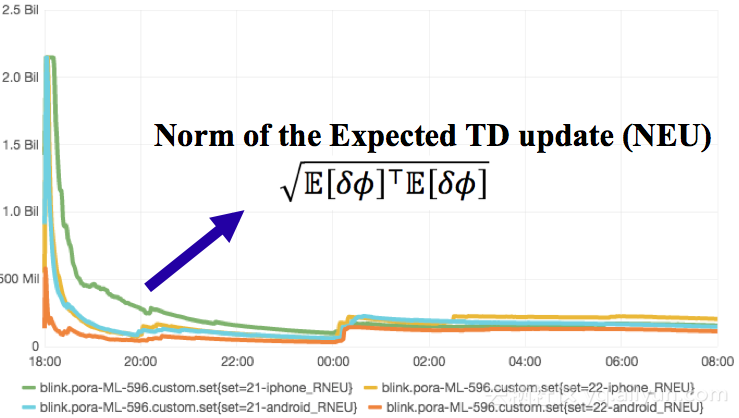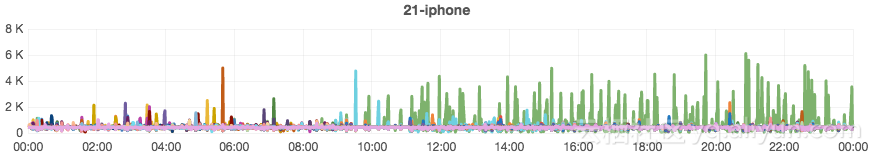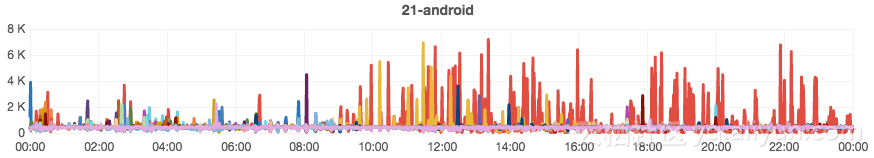### DDPG与梯度融合

$$L(a,X,Y) = \frac{1}{n}\sum_i^n y_i \log(\sigma(a^T x_i)) + (1-y_i)\log(1-\sigma(a^T x_i))$$

$$\nabla_{\theta^{\mu}} J = - \frac{1}{N} \sum_i^N \nabla_a Q(s,a|\theta^Q)|_{s=s_i,a=\mu(s_i)}\nabla_{\theta^{\mu}} \mu(s|\theta^{\mu})|_{s_i} + \nonumber$$

$$\lambda \nabla_a L(a,X,Y)|_{a=\mu(s_i)}\nabla_{\theta^{\mu}} \mu(s|\theta^{\mu})|_{s_i}$$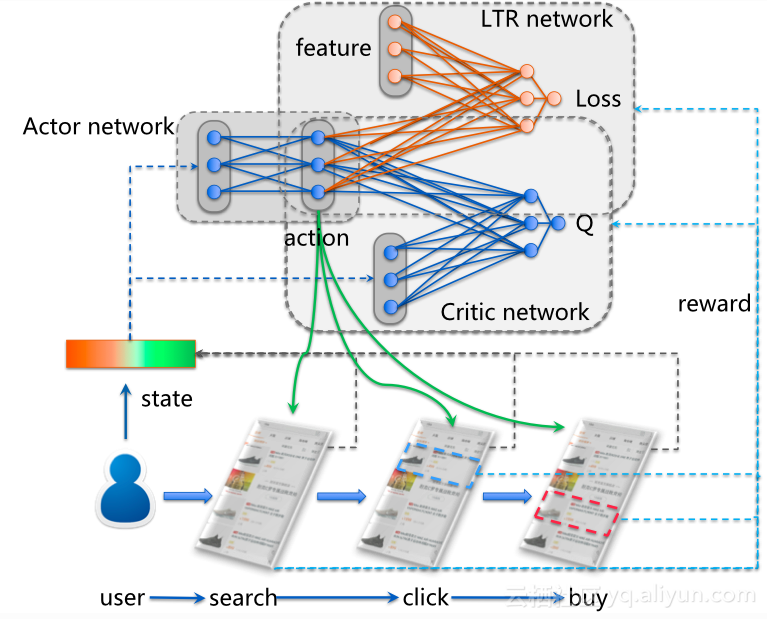## 总结与展望

（1）状态的表示：我们将用户最近点击的商品特征和用户长期行为特征作为状态，其实是基于这样的一个假设，即用户点击过的商品能够较为精确地反映用户的内心活动和对商品的偏好。但实际上，用户对商品的点击通常具有盲目性，无论什么商品可能都想要看一看。也就是说，我们凭借经验所设定的状态并非那么准确。深度强化学习对状态特征的自动抽取能力是它在Atari Game和围棋上取得成功的重要原因之一。因此，在短期内可以考虑利用深度强化学习对现有方案进行扩展。同时，借助深度神经网络对状态特征的自动抽取，我们也可以发现用户的哪些行为对于搜索引擎的决策是比较重要的。

（2）奖赏函数的设定：和状态的定义一样，我们在第二章设定的奖赏函数也来自于人工经验。奖赏塑形（Reward Shaping）虽然是优化奖赏函数的方法，但其本质上也是启发式函数，其更多的作用在于对学习算法的加速。逆强化学习（Inverse Reinforcement Learning，IRL）是避免人工设定的奖赏函数的有效途径之一，也是强化学习研究领域的重要分支。IRL的主要思想是根据已知的专家策略或行为轨迹，通过监督学习的方法逆推出问题模型的奖赏函数。Agent在这样的奖赏函数上进行学习，就能还原出专家策略。对于我们的问题，IRL的现有方法不能完全适用，因为我们的搜索任务并不存在一个可供模仿的专家策略。我们需要更深入思考如何在奖赏函数与我们的目标（提升CTR，提升成交笔数）之间建立紧密的关系。

（3）多智能体强化学习（Multi-Agent Reinforcement Learning, MARL）：我们将搜索引擎看作Agent，把用户看成响应Agent动作的环境，属于典型的单智能体强化学习（Single-Agent RL）模式。在单智能体强化学习的理论模型（即MDP）中，环境动态（Environmental Dynamics，也即奖赏函数和状态转移函数）是不会发生变化的；而在我们的问题中，用户的响应行为却是非静态的，同时也带有随机性。因此，单智能体强化学习的模式未必是我们的最佳方案。要知道，用户其实也是在一定程度理性控制下的，能够进行自主决策甚至具有学习能力的Agent。从这样的视角来看，或许更好的方式是将用户建模为另外一个Agent，对这个Agent的行为进行显式地刻画，并通过多智能体强化学习方法来达到搜索引擎Agent和用户Agent之间的协同（Coordination）。

（4）第四章的末尾提到了奖赏函数与Agent的动作的相互作用带来的非独立同分布数据问题，我们在这里再进行一些讨论。在MDP模型中，奖赏函数$R(s,a,s')$（有时又写成$R(s,a)$）是固定的，不会随着Agent策略的变化而变化。然而，在我们提出的奖赏塑形方法中，势函数$\Phi_{clk}(s)$和$\Phi_{pay}(s)$中包含了策略参数$\theta$，使得Agent从环境获得的奖赏信号在不同的$\theta$下有所不同。这也意味着我们的Agent实际上是处于一个具有动态奖赏函数的环境中，这种动态变化不是来自于外部环境，而是源于Agent的策略改变。这有点类似于人的行为与世界的相互作用。这样一来，我们可以将3.2节的$J(\mu_{\theta})$重写为

$$\begin{split} \bar{J}(\mu_{\theta}) &= \int_S \int_S \sum_{t=1}^{\infty} \gamma^{t-1} p_0(s') T(s', \mu_{\theta}(s'), s) R_{\theta}(s, \mu_{\theta}(s)) \text{ d}s' \text{ d}s \\ &= \int_S \rho^{\mu}(s) R_{\theta}(s, \mu_{\theta}) \text{ d}s. \end{split}$$

# 强化学习为何有用？——延迟奖赏在搜索排序场景中的作用分析 （哲予）

## 一、 背景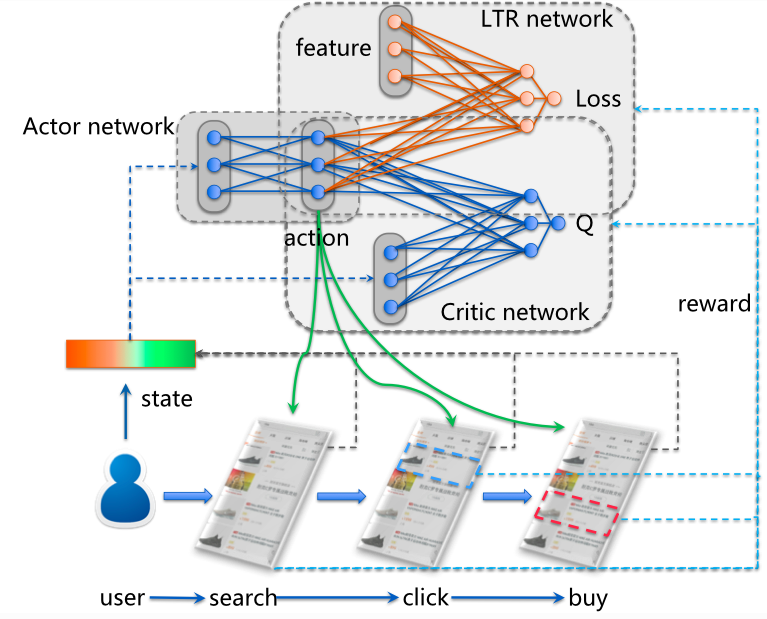## 二、搜索排序问题回顾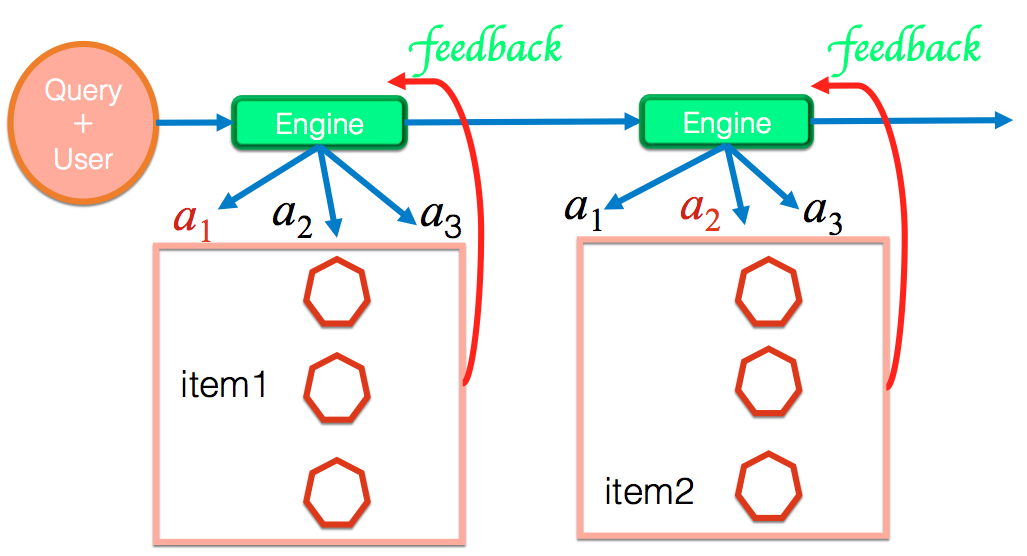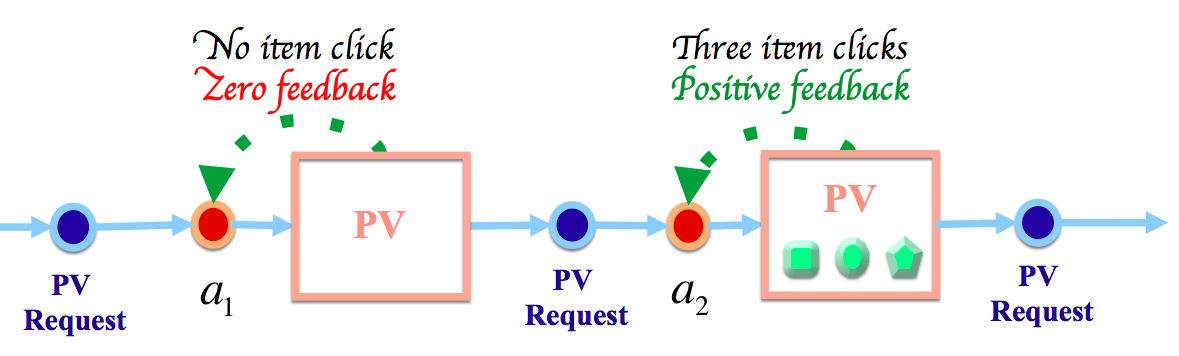## 三、数据统计分析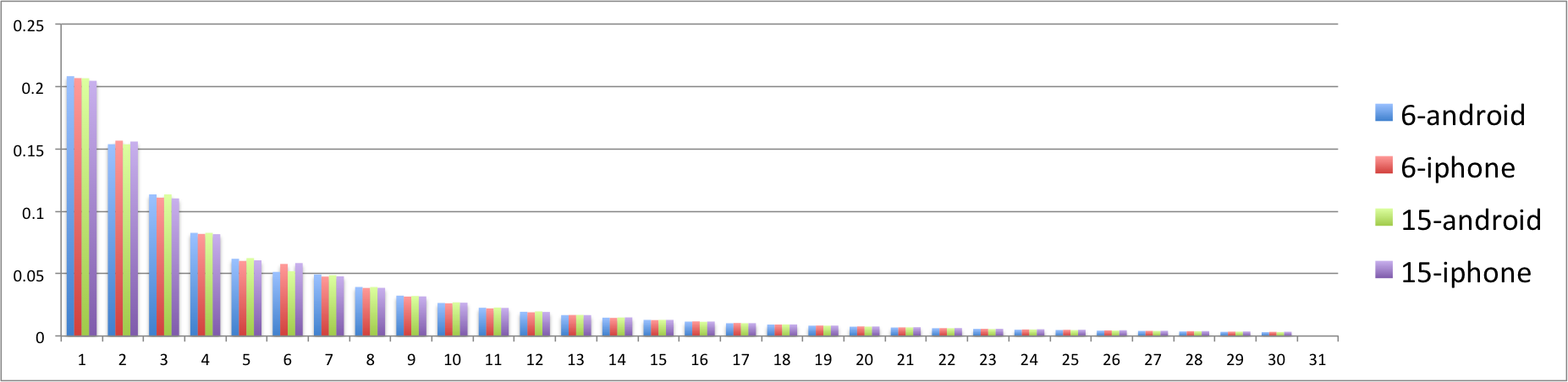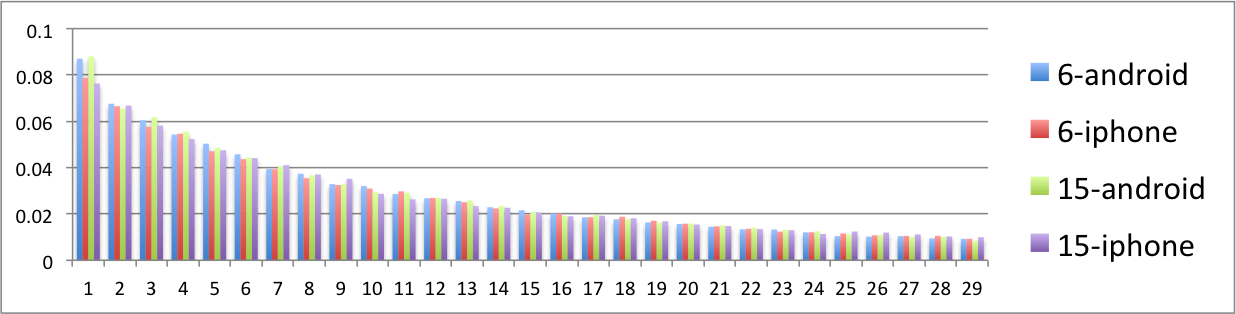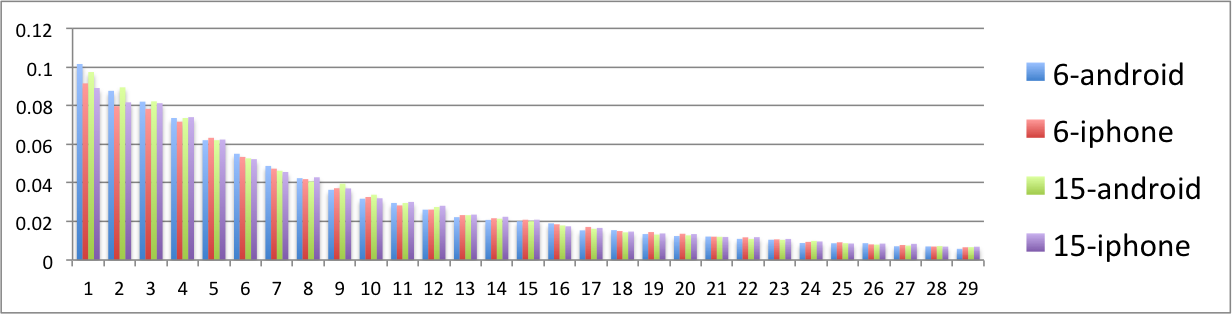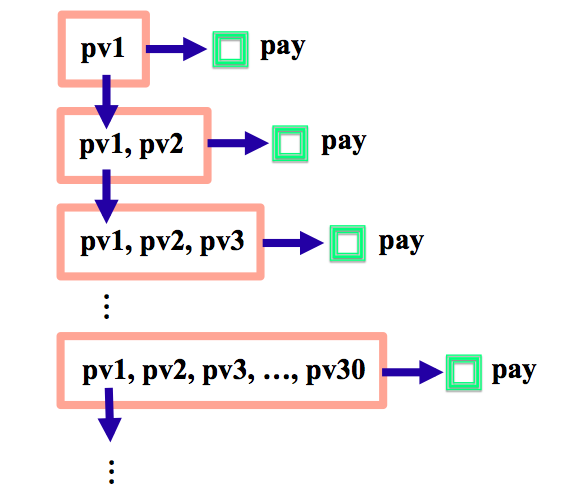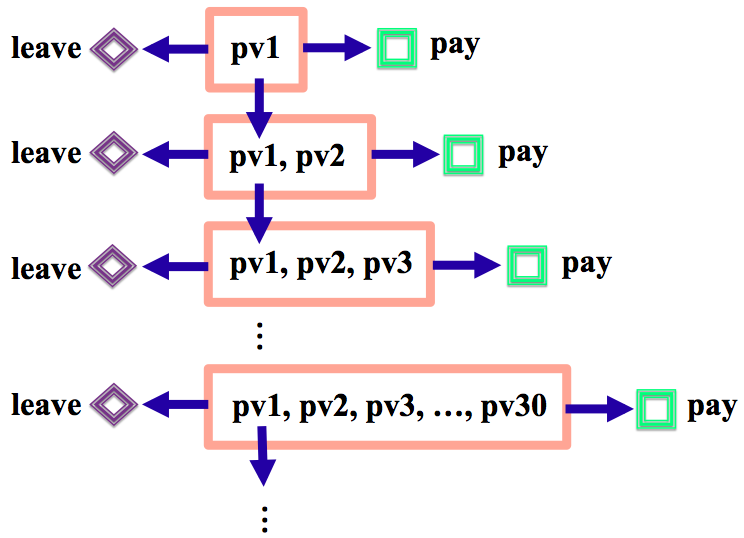## 四、搜索排序问题形式化

• $T = \lceil \frac{|\mathcal{D}|}{K} \rceil$为搜索会话最大决策步数,
• $\mathcal{H} = \bigcup_{t=0}^T \mathcal{H}_t$为关于$q$、$\mathcal{D}$和$K$的所有可能的item page history的集合，其中$\mathcal{H}_t$为$t$时刻所有可能item page history的集合($0 \leq t \leq T$)，
• $\mathcal{S} = \mathcal{H}_C \bigcup \mathcal{H}_B \bigcup \mathcal{H}_L$为状态空间，$\mathcal{H}_C = \{ C(h_t) | \forall h_t \in \mathcal{H}_t, 0 \leq t < T \}$是包含所有的继续会话事件的非终止状态集合（nonterminal state set），$\mathcal{H}_B = \{ B(h_t) | \forall h_t \in \mathcal{H}_t, 0 < t \leq T \}$和$\mathcal{H}_L = \{ L(h_t) | \forall h_t \in \mathcal{H}_t, 0 < t \leq T \}$分别是包含所有成交转化事件离开事件的终止状态集合（terminal state set），
• $\mathcal{A}$为动作空间，包含搜索引擎所有可能的排序打分函数，
• $\mathcal{R} : \mathcal{H}_C \times \mathcal{A} \times \mathcal{S} \rightarrow \mathbb{R}$为奖赏函数，
• $\mathcal{P} : \mathcal{H}_C \times \mathcal{A} \times \mathcal{S} \rightarrow [0,1]$为状态转移函数，对于任意时间步$t$（$0 \leq t < T$）、任意item page history $h_t \in \mathcal{H}_t$和任意动作$a \in \mathcal{A}$，令$h_{t+1} = (h_t, \mathcal{L}_K(\mathcal{D}_t, a))$，则agent在状态$C(h_t)$上执行动作$a$后，环境转移到任意状态$s' \in \mathcal{S}$的概率为

\mathcal{P}(C(h_t), a, s') = \left\{ \begin{aligned} &b(h_{t+1}) &\text{if } s' = B(h_{t+1}), \\ &l(h_{t+1}) &\text{if } s' = L(h_{t+1}), \\ &c(h_{t+1}) &\text{if } s' = C(h_{t+1}), \\ &0 &\text{otherwise.} \end{aligned} \right.

\mathcal{R}(C(h_t), a, s') = \left\{ \begin{aligned} &m(h_{t+1}) &\text{ if } s' = B(h_{t+1}), \\ &0 &\text{otherwise,} \quad\quad \end{aligned} \right.

## 五、理论分析

### 5.1 马尔可夫性质

$$\text{Pr}(s_t | s_0, a_0, s_1, a_1, ..., s_{t-1}, a_{t-1}) = \text{Pr}(s_t | s_{t-1}, a_{t-1}).$$

$$h_{t'} = (h_{t' - 1}, \mathcal{L}_K(\mathcal{D}_{t'-1}, a_{t'-1}))$$

$$\begin{split} &\text{Pr}(s_t | s_0, a_0, s_1, a_1, ..., s_{t-1}, a_{t-1}) \\ = &\text{Pr}(s_t | C(h_0), a_0, C(h_1), a_1, ..., C(h_{t-1}), a_{t-1}) \\ = &\text{Pr}(s_t | h_1, h_2, ..., h_{t-1}, C(h_{t-1}), a_{t-1}) \\ = &\text{Pr}(s_t | h_{t-1}, C(h_{t-1}), a_{t-1}) \\ = &\text{Pr}(s_t | C(h_{t-1}), a_{t-1}) \\ = &\text{Pr}(s_t | s_{t-1}, a_{t-1}). \end{split}$$

### 5.2 折扣率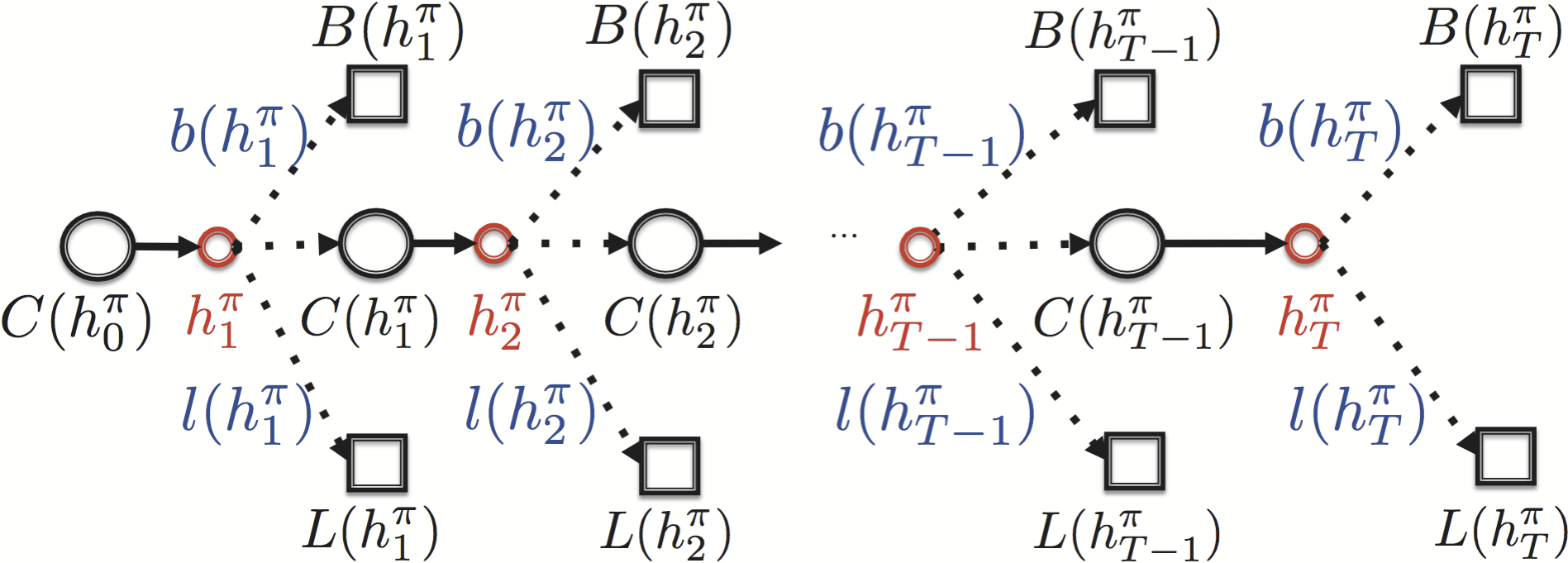$$\begin{split} V^{\pi}_{\gamma}(C^{\pi}_t) &= \mathbb{E}^{\pi} \big\\{ \sum_{k=1}^{T-t} \gamma^{k-1} r_{t+k} \big| C^{\pi}_t \big\\} \\ &= \mathbb{E}^{\pi} \big\\{ r_{t+1} + \gamma r_{t+2} + \cdot\cdot\cdot + \gamma^{T-t-1} r_{T} \big| C^{\pi}_t \big\\}. \end{split}$$

\text{Pr}(C^{\pi}_t \rightarrow h^{\pi}_{t+k}) = \left\{ \begin{aligned} 1.0 &k = 1, \quad\quad\quad \\ \Pi^{k-1}_{j=1} c^{\pi}_{t+j} &1 < k \leq T-t. \end{aligned} \right.

$$\begin{split} V^{\pi}_{\gamma}(C^{\pi}_t) &= \mathbb{E}^{\pi} \big\\{ r_{t+1} \big| C^{\pi}_t \big\\} + \gamma \mathbb{E}^{\pi} \big\\{r_{t+2} \big| C^{\pi}_t \big\\} + \cdot\cdot\cdot + \gamma^{T-t-1} \mathbb{E}^{\pi} \big\\{ r_{T} \big| C^{\pi}_t \big\\} \\ &= \sum^{T-t}_{k=1} \gamma^{k-1} \text{Pr}(C^{\pi}_t \rightarrow h^{\pi}_{t+k}) b^{\pi}_{t+k} m^{\pi}_{t+k} \\ &= b^{\pi}_{t+1} m^{\pi}_{t+1} + \gamma c^{\pi}_{t+1} b^{\pi}_{t+2} m^{\pi}_{t+2} + \cdot\cdot\cdot + \gamma^{T-t-1} \big( \Pi^{T-t-1}_{j=1} c^{\pi}_{t+j} \big) b^{\pi}_{T} m^{\pi}_{T} \\ &= b^{\pi}_{t+1} m^{\pi}_{t+1} + \sum^{T-t}_{k=2} \gamma^{k-1} \Big( \big( \Pi^{k-1}_{j=1} c^{\pi}_{t+j} \big) b^{\pi}_{t+k} m^{\pi}_{t+k} \Big). \end{split}$$

$$\begin{split} \mathbb{E}^{\pi}_{gmv} &= b^{\pi}_{1} m^{\pi}_1 + c^{\pi}_1 b^{\pi}_2 m^{\pi}_2 + \cdot\cdot\cdot + \big( \Pi_{k=1}^{T} c^{\pi}_k \big) b^{\pi}_T m^{\pi}_T \\ &= b^{\pi}_{1} m^{\pi}_1 + \sum_{k=2}^{T} \big( \Pi_{j=1}^{k-1} c^{\pi}_j \big) b^{\pi}_k m^{\pi}_k. \end{split}$$

# 六. 实验分析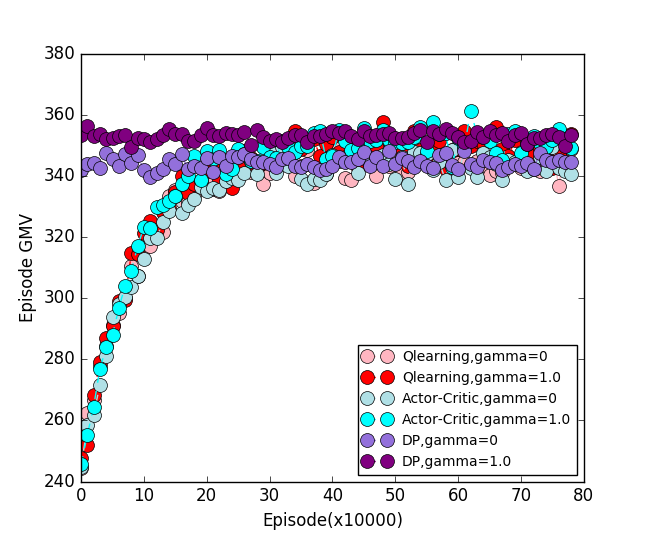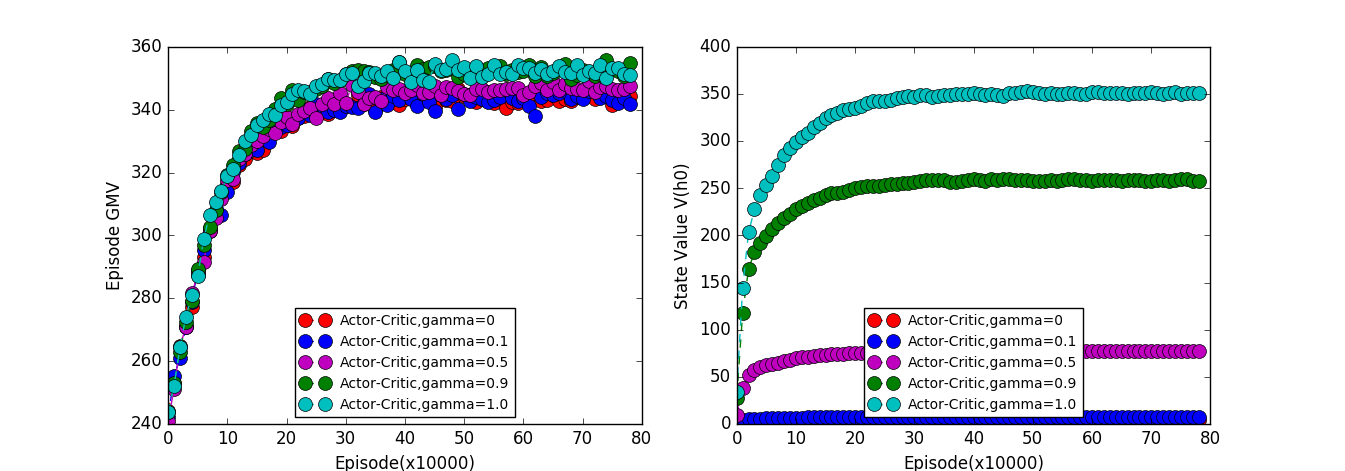# 引擎性能优化

## 一、背景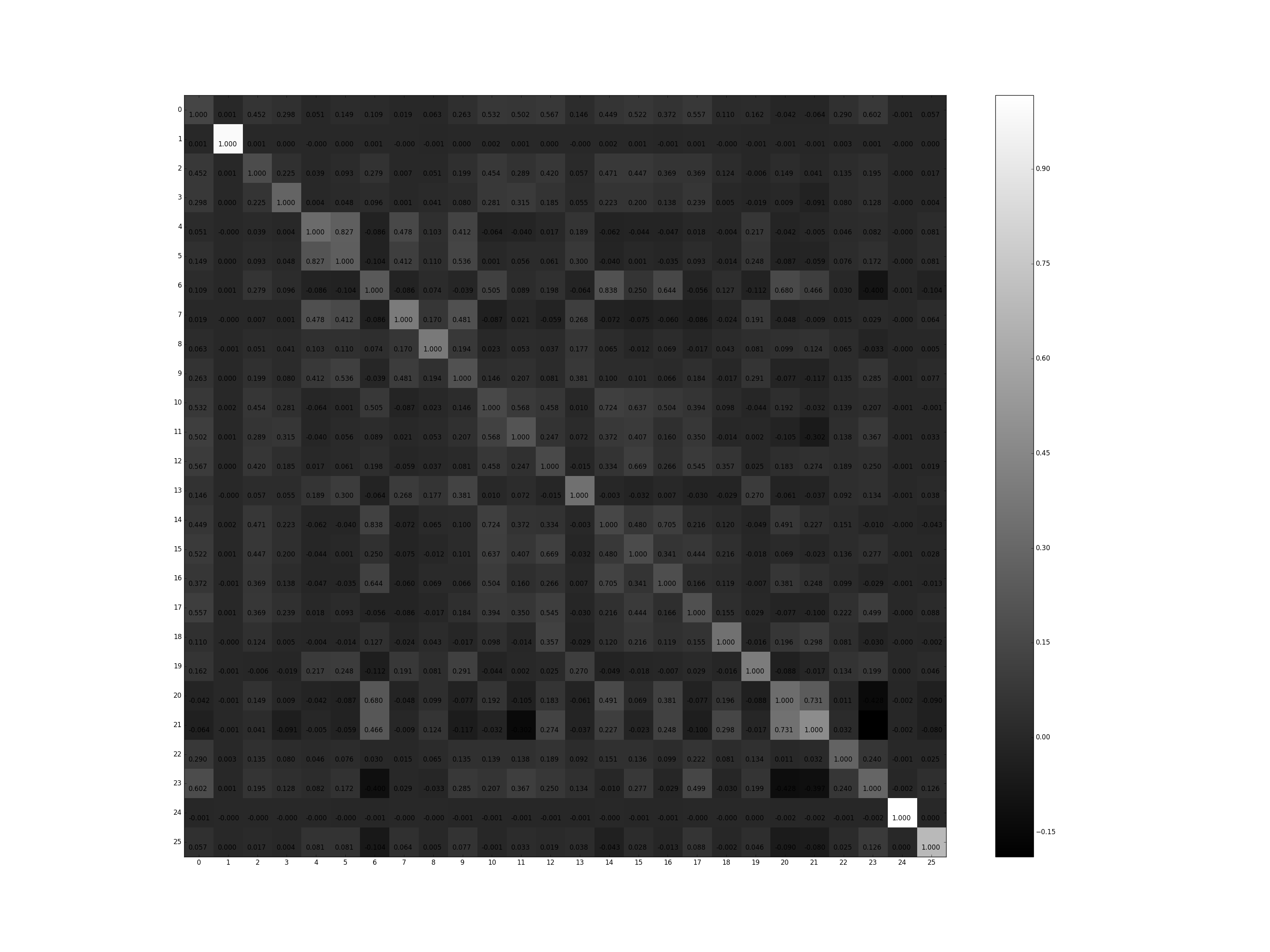## 二、问题建模

\begin{align} F(u,q,d)=f\big(x_{1}(d),x_{2}(d),...,x_{n}(d)\big) \end{align}

\begin{align} \min_{S \subset \Omega} \;\; D(F_o||F_a) + \lambda ||S||_0 \end{align}

\begin{align} S_{u,q}=H(u,q|\theta) \end{align}### 2.1 状态定义

$$s = (age, sex, power, a_1, a_2,\dots,a_n,current\text{_}step)$$

### 2.3 状态转移函数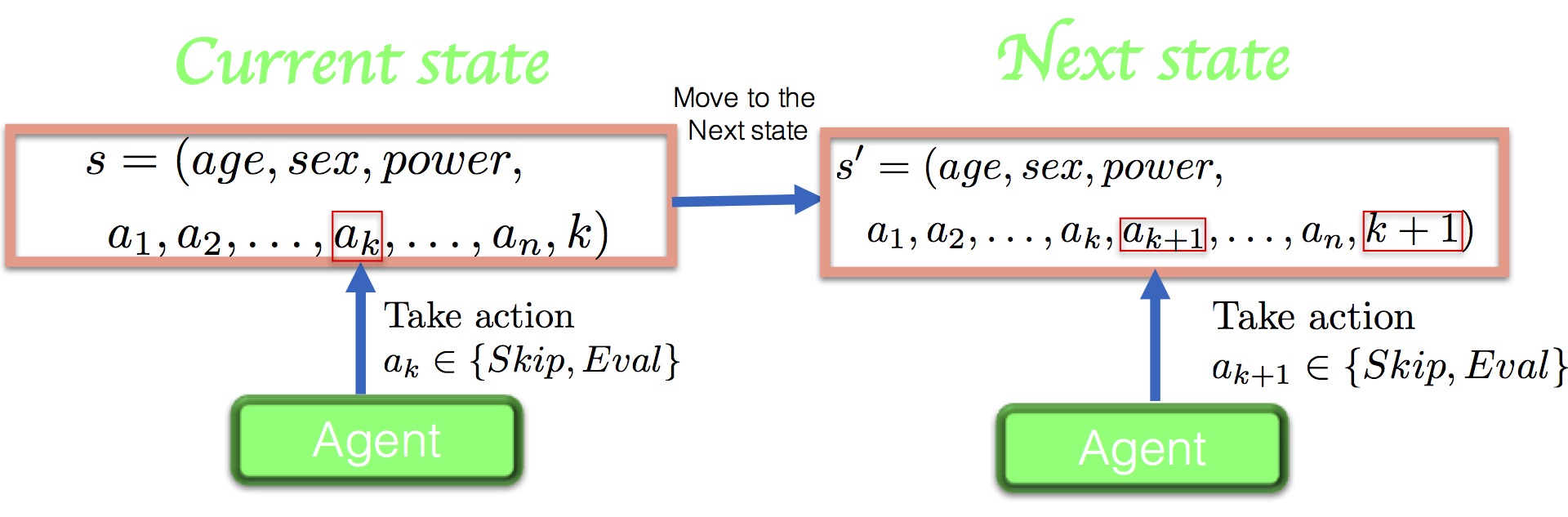agent根据当前状态$s$, 做出决策，选择动作$a_k$；这时将动作$a_k$存储在$s^\prime$中，同时最后一维计数$k+1$。重复以上过程直到最后一维到达某个值。

### 2.4 奖赏函数的设计

$$b(a_k) = \begin{cases} 0 &\text{if a_k= Skip}\\ 1 &\text{if a_k= Eval} \end{cases}$$

### 3.3 算法设计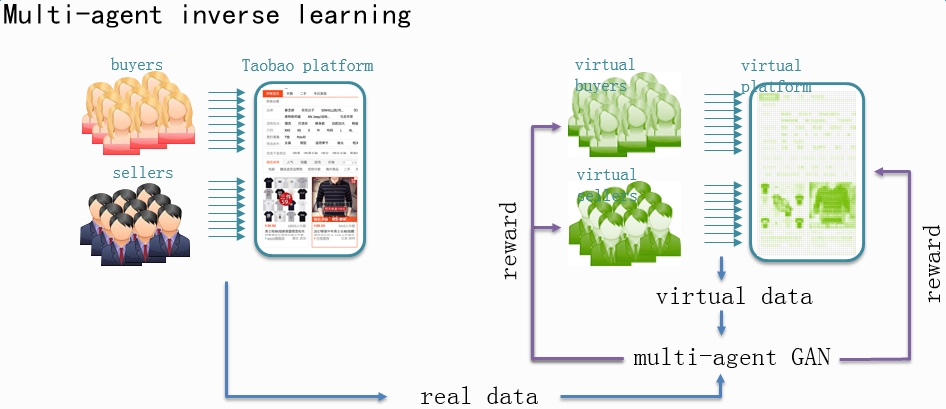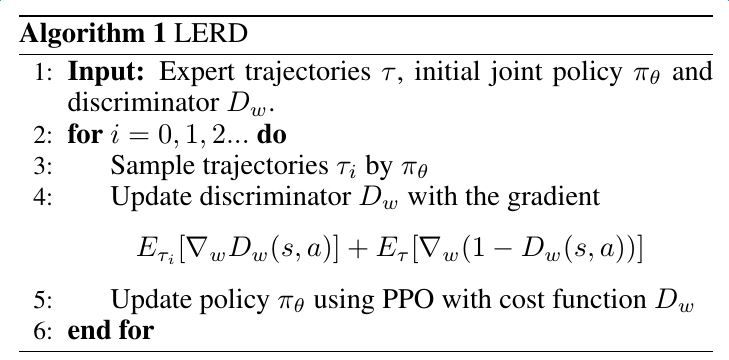# 致谢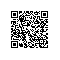AI Online Serving，阿里巴巴集团搜索与推荐，算法与工程技术的大本营，大数据深度学习时代的创新主场。